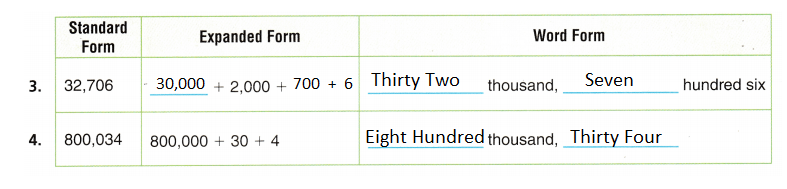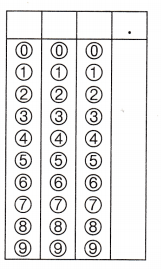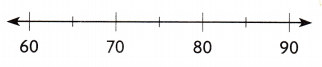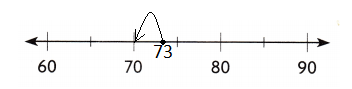Refer to our Texas Go Math Grade 3 Answer Key Pdf to score good marks in the exams. Test yourself by practicing the problems from Texas Go Math Grade 3 Module 1 Assessment Answer Key.

Vocabulary

Choose the best term from the box to complete the sentence.

Question 1.
The ___ of a number is a way to write a number by showing the value of each digit.

The Expanded Notation of a number is a way to write a number by showing the value of each digit.

Question 2.
The ___ of a number is a way to write a number by using words.

The Numeration of a number is a way to write a number by using words.

Concepts and Skills

Complete the chart to show three forms of the number. TEKS 3.2.ARound to the nearest hundred.

Question 5.
409 ____

The nearest Hundred for 409 is 400

Question 6.
876 ___

The nearest Hundred for 876 is 900

Question 7.
399 ____

The nearest Hundred for 399 is 400

Round to the nearest thousand. TEKS 3.2C

Question 8.
4,397 ____

The nearest thousand for 4,379 is 4,440

Question 9.
8,222 ____

The nearest Thousand for 8,222 is 8,200

Question 10.
7,656 ____

The Nearest Thousand for 7,656 is 7,700.

Compare the numbers. Write <, >, or in the.

Question 11.
8915,902

891 < 5,902

Question 12.
6,8126,812

6,812 = 6,812

Question 13.
18,00117,897

18,001 > 17,897

Texas Test prep

Fill in the bubble for the correct answer choice. You may use models to solve.

Question 14.
An order for 875,380 toys is being shipped to a warehouse in the United States. How many ten thousand’s are in 875,380?
(A) 8
(B) 87
(C) 875
(D) 8,753

(B) 87 is the correct answer choice.

Because

0 in Ones Place

8 in Tens Place

3 in Hundreds Place

5 in Thousands Place

7 in Ten Thousand Place

8 in Lakhs Place

So, 87 ten thousand are in 875,380.

Question 15.
There were 4,619 students enrolled in a military service academy in Colorado. Which is the number of students
written in expanded form? TEKS 3.2.A
(A) 4,000 + 600 + 10 + 9
(B) 9,000 + 100 + 60 + 4
(C) 400 + 600 + 10 + 9
(D) 4,000 + 600 + 9

(A) 4,000 + 600 + 10 + 9 is the Corect answer choice

Because, 4,000 + 600 + 10 + 9 = 4,619 is the total students enrolled in a military service academy in Colorado.

Question 16.
One killer whale at the Sea Center weighed 9,485 pounds. Which is a way to model 9,485 with base-ten blocks. TEKS 3.2.A
(A) 9 thousand 48 hundred 5 ones
(B) 94 thousand 85 ones
(C) 9,485 tens
(D) 9 thousand 48 tens 5 ones

(A) 9 thousand 48 hundred 5 ones

Because,  9 thousand 48 hundred 5 ones = 9000 + 480 + 5 = 9,485 pounds.

Question 17.
Alexis rounded 73 to the nearest ten. What is 73 rounded to the nearest ten? TEKS 3.2.C.